# Change the data type of a column or a Pandas Series

Series is a one-dimensional labeled array capable of holding data of the type integer, string, float, python objects, etc. The axis labels are collectively called index.

Let’s see the program to change the data type of column or a Series in Pandas Dataframe.
Method 1: Using DataFrame.astype() method.

We can pass any Python, Numpy or Pandas datatype to change all columns of a dataframe to that type, or we can pass a dictionary having column names as keys and datatype as values to change type of selected columns.

Syntax: DataFrame.astype(dtype, copy = True, errors = ’raise’, **kwargs)

Return: casted : type of caller

Let’s see the examples:
Example 1: The Data type of the column is changed to “str” object.

## Python3

 `# importing the pandas library ` `import` `pandas as pd ` ` `  `# creating a DataFrame ` `df ``=` `pd.DataFrame({``'srNo'``: [``1``, ``2``, ``3``], ` `                   ``'Name'``: [``'Geeks'``, ``'for'``,  ` `                            ``'Geeks'``],  ` `                   ``'id'``: [``111``, ``222``,  ` `                          ``333``]}) ` `# show the dataframe ` `print``(df) ` ` `  `# show the datatypes ` `print``(df.dtypes)`

Output: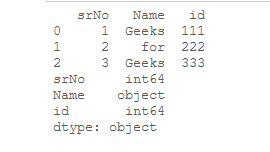Now, changing the dataframe data types to string.

## Python3

 `# changing the dataframe  ` `# data types to string ` `df ``=` `df.astype(``str``) ` ` `  `# show the data types  ` `# of dataframe ` `df.dtypes`

Output: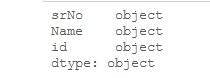Example 2: Now, let us change the data type of the “id” column from “int” to “str”. We create a dictionary and specify the column name with the desired data type.

## Python3

 `# importing the pandas library ` `import` `pandas as pd ` ` `  `# creating a DataFrame ` `df ``=` `pd.DataFrame({``'No'``: [``1``, ``2``, ``3``], ` `                   ``'Name'``: [``'Geeks'``, ``'for'``,  ` `                            ``'Geeks'``],  ` `                   ``'id'``: [``111``, ``222``,  ` `                          ``333``]}) ` `# show the dataframe ` `print``(df) ` ` `  `# show the datatypes ` `print``(df.dtypes)`

Output: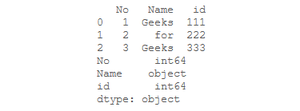Now, change the data type of ‘id’ column to string.

## Python3

 `# creating a dictionary  ` `# with column name and data type ` `data_types_dict ``=` `{``'id'``: ``str``} ` ` `  `# we will change the data type  ` `# of id column to str by giving ` `# the dict to the astype method ` `df ``=` `df.astype(data_types_dict) ` ` `  `# checking the data types ` `# using df.dtypes method ` `df.dtypes`

Output:Example 3: Convert the data type of “grade” column from “float” to “int”.

## Python3

 `# import pandas library ` `import` `pandas as pd ` ` `  `# dictionary ` `result_data ``=` `{``'name'``: [``'Alia'``, ``'Rima'``, ``'Kate'``, ` `                        ``'John'``, ``'Emma'``, ``'Misa'``, ` `                        ``'Matt'``], ` `        ``'grade'``: [``13.5``, ``7.1``, ``11.5``,  ` `                  ``3.77``, ``8.21``, ``21.22``, ` `                  ``17.5``], ` `        ``'qualify'``: [``'yes'``, ``'no'``, ``'yes'``, ` `                    ``'no'``, ``'no'``, ``'yes'``,  ` `                    ``'yes'``]} ` ` `  `# create a dataframe ` `df ``=` `pd.DataFrame(result_data) ` ` `  `# show the dataframe ` `print``(df) ` ` `  `#show the datatypes ` `print``(df.dtypes)`

Output: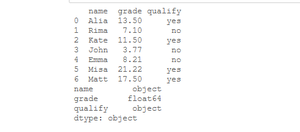Now, we convert the data type of “grade” column from “float” to “int”.

## Python3

 `# convert data type of grade column  ` `# into integer ` `df.grade ``=` `df.grade.astype(``int``) ` ` `  `# show the dataframe ` `print``(df) ` ` `  `# show the datatypes ` `print``(df.dtypes)`

Output: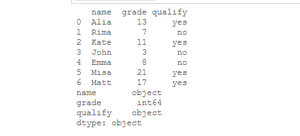Method 2: Using Dataframe.apply() method.

We can pass pandas.to_numeric, pandas.to_datetime and pandas.to_timedelta as argument to apply() function to change the datatype of one or more columns to numeric, datetime and timedelta respectively.

Syntax: Dataframe/Series.apply(func, convert_dtype=True, args=())

Return: Dataframe/Series after applied function/operation.

Let’s see the example:

Example: Convert the data type of “B” column from “string” to “int”.

## Python3

 `# importing pandas as pd  ` `import` `pandas as pd  ` `   `  `# sample dataframe  ` `df ``=` `pd.DataFrame({  ` `    ``'A'``: [``'a'``, ``'b'``, ``'c'``,  ` `          ``'d'``, ``'e'``],  ` `    ``'B'``: [``12``, ``22``, ``35``, ` `          ``'47'``, ``'55'``],  ` `    ``'C'``: [``1.1``, ``'2.1'``, ``3.0``, ` `          ``'4.1'``, ``'5.1'``] })  ` ` `  `# show the dataframe ` `print``(df) ` ` `  `# show the data types ` `# of all columns ` `df.dtypes`

Output: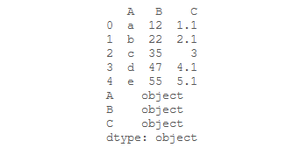Now, we convert the datatype of column “B” into an “int” type.

## Python3

 `# using apply method  ` `df[[``'B'``]] ``=` `df[[``'B'``]].``apply``(pd.to_numeric)  ` ` `  `# show the data types ` `# of all columns ` `df.dtypes`

Output: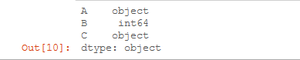My Personal Notes arrow_drop_upGeeksforGeeks Intern

If you like GeeksforGeeks and would like to contribute, you can also write an article using contribute.geeksforgeeks.org or mail your article to contribute@geeksforgeeks.org. See your article appearing on the GeeksforGeeks main page and help other Geeks.

Please Improve this article if you find anything incorrect by clicking on the "Improve Article" button below.

Article Tags :

Be the First to upvote.

Please write to us at contribute@geeksforgeeks.org to report any issue with the above content.